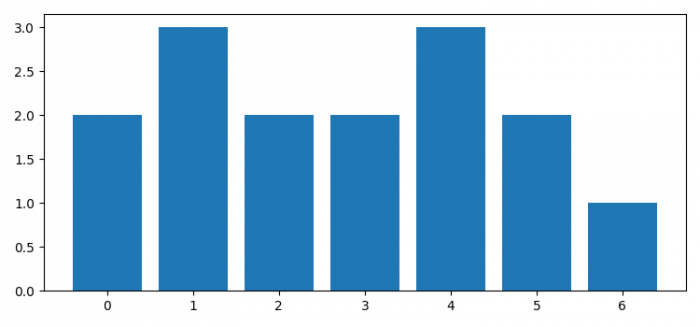# How to plot collections.Counter histogram using Matplotlib?

To plot a histogram, with collections.Counter, we can use bar() method. In bar() method, we can use collections.counter() to get the frequency for each element. Put the elements and their frequency as height.

## Steps

• Set the figure size and adjust the padding between and around the subplots.
• Make a list of a data points.
• Get the dictionary, d, using collections.Counter().
• Make bar plot with d.keys() and d.values().
• To display the figure, use show() method.

## Example

import collections
from matplotlib import pyplot as plt

plt.rcParams["figure.figsize"] = [7.50, 3.50]
plt.rcParams["figure.autolayout"] = True

data = [0, 1, 2, 4, 1, 3, 0, 4, 1, 4, 3, 5, 6, 5, 2]

d = collections.Counter(data)

plt.bar(d.keys(), d.values())

plt.show()

## Output